9 Linear Momentum and Collisions

# 9.2 Impulse and Collisions

### Learning Objectives

By the end of this section, you will be able to:

• Explain what an impulse is, physically
• Describe what an impulse does
• Relate impulses to collisions
• Apply the impulse-momentum theorem to solve problems

We have defined momentum to be the product of mass and velocity. Therefore, if an object’s velocity should change (due to the application of a force on the object), then necessarily, its momentum changes as well. This indicates a connection between momentum and force. The purpose of this section is to explore and describe that connection.

Suppose you apply a force on a free object for some amount of time. Clearly, the larger the force, the larger the object’s change of momentum will be. Alternatively, the more time you spend applying this force, again the larger the change of momentum will be, as depicted in Figure. The amount by which the object’s motion changes is therefore proportional to the magnitude of the force, and also to the time interval over which the force is applied.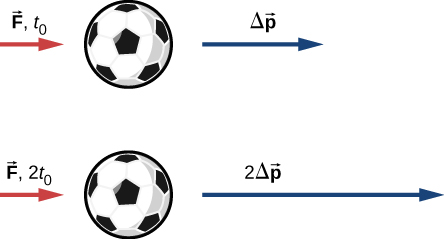Figure 9.5 The change in momentum of an object is proportional to the length of time during which the force is applied. If a force is exerted on the lower ball for twice as long as on the upper ball, then the change in the momentum of the lower ball is twice that of the upper ball.

Mathematically, if a quantity is proportional to two (or more) things, then it is proportional to the product of those things. The product of a force and a time interval (over which that force acts) is called impulse, and is given the symbol $\mathbf{\overset{\to }{J}}.$

#### Impulse

Let $\mathbf{\overset{\to }{F}}(t)$ be the force applied to an object over some differential time interval dt (Figure). The resulting impulse on the object is defined as

$d\mathbf{\overset{\to }{J}}\equiv \mathbf{\overset{\to }{F}}(t)dt.$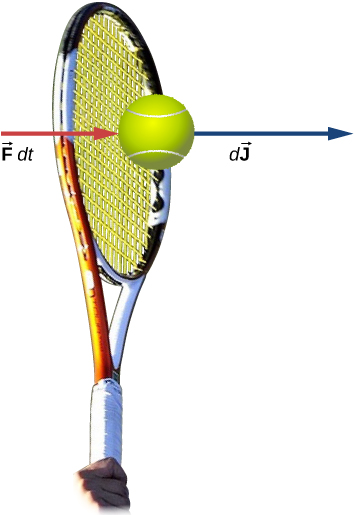Figure 9.6 A force applied by a tennis racquet to a tennis ball over a time interval generates an impulse acting on the ball.

The total impulse over the interval ${t}_{\text{f}}-{t}_{\text{i}}$ is

$\mathbf{\overset{\to }{J}}={\int }_{{t}_{\text{i}}}^{{t}_{\text{f}}}d\mathbf{\overset{\to }{J}}\enspace\text{or}\enspace\mathbf{\overset{\to }{J}}\equiv {\int }_{{t}_{\text{i}}}^{{t}_{\text{f}}}\mathbf{\overset{\to }{F}}(t)dt.$

Figure and Figure together say that when a force is applied for an infinitesimal time interval dt, it causes an infinitesimal impulse $d\mathbf{\overset{\to }{J}}$, and the total impulse given to the object is defined to be the sum (integral) of all these infinitesimal impulses.

To calculate the impulse using Figure, we need to know the force function F(t), which we often don’t. However, a result from calculus is useful here: Recall that the average value of a function over some interval is calculated by

$f{(x)}_{\text{ave}}=\frac{1}{\Delta x}{\int }_{{x}_{\text{i}}}^{{x}_{\text{f}}}f(x)dx$

where $\Delta x={x}_{\text{f}}-{x}_{\text{i}}$. Applying this to the time-dependent force function, we obtain

${\mathbf{\overset{\to }{F}}}_{\text{ave}}=\frac{1}{\Delta t}{\int }_{{t}_{\text{i}}}^{{t}_{\text{f}}}\mathbf{\overset{\to }{F}}(t)dt.$

Therefore, from Figure,

$\mathbf{\overset{\to }{J}}={\mathbf{\overset{\to }{F}}}_{\text{ave}}\Delta t.$

The idea here is that you can calculate the impulse on the object even if you don’t know the details of the force as a function of time; you only need the average force. In fact, though, the process is usually reversed: You determine the impulse (by measurement or calculation) and then calculate the average force that caused that impulse.

To calculate the impulse, a useful result follows from writing the force in Figure as $\mathbf{\overset{\to }{F}}(t)=m\mathbf{\overset{\to }{a}}(t)$:

$\mathbf{\overset{\to }{J}}={\int }_{{t}_{\text{i}}}^{{t}_{\text{f}}}\mathbf{\overset{\to }{F}}(t)dt=m{\int }_{{t}_{\text{i}}}^{{t}_{\text{f}}}\mathbf{\overset{\to }{a}}(t)dt=m[\mathbf{\overset{\to }{v}}({t}_{\text{f}})-{\mathbf{\overset{\to }{v}}}_{\text{i}}].$

For a constant force ${\mathbf{\overset{\to }{F}}}_{\text{ave}}=\mathbf{\overset{\to }{F}}=m\mathbf{\overset{\to }{a}}$, this simplifies to

$\mathbf{\overset{\to }{J}}=m\mathbf{\overset{\to }{a}}\Delta t=m{\mathbf{\overset{\to }{v}}}_{\text{f}}-m{\mathbf{\overset{\to }{v}}}_{\text{i}}=m({\mathbf{\overset{\to }{v}}}_{\text{f}}-{\mathbf{\overset{\to }{v}}}_{\text{i}}).$

That is,

$\mathbf{\overset{\to }{J}}=m\Delta \mathbf{\overset{\to }{v}}.$

Note that the integral form, Figure, applies to constant forces as well; in that case, since the force is independent of time, it comes out of the integral, which can then be trivially evaluated.

### Example

#### The Arizona Meteor Crater

Approximately 50,000 years ago, a large (radius of 25 m) iron-nickel meteorite collided with Earth at an estimated speed of $1.28\times {10}^{4}\,\text{m/s}$ in what is now the northern Arizona desert, in the United States. The impact produced a crater that is still visible today (Figure); it is approximately 1200 m (three-quarters of a mile) in diameter, 170 m deep, and has a rim that rises 45 m above the surrounding desert plain. Iron-nickel meteorites typically have a density of $\rho =7970{\,\text{kg/m}}^{3}$. Use impulse considerations to estimate the average force and the maximum force that the meteor applied to Earth during the impact.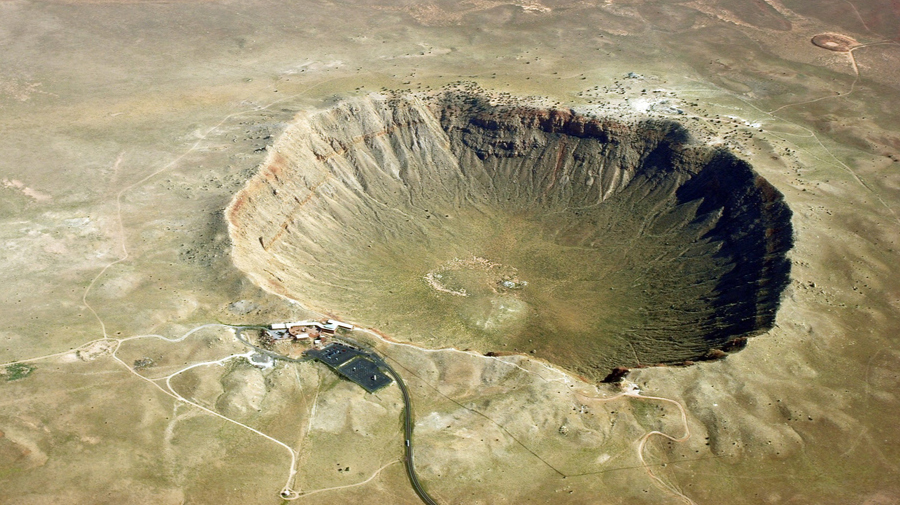Figure 9.7 The Arizona Meteor Crater in Flagstaff, Arizona (often referred to as the Barringer Crater after the person who first suggested its origin and whose family owns the land). (credit: “Shane.torgerson”/Wikimedia Commons)

#### Strategy

It is conceptually easier to reverse the question and calculate the force that Earth applied on the meteor in order to stop it. Therefore, we’ll calculate the force on the meteor and then use Newton’s third law to argue that the force from the meteor on Earth was equal in magnitude and opposite in direction.

Using the given data about the meteor, and making reasonable guesses about the shape of the meteor and impact time, we first calculate the impulse using Figure. We then use the relationship between force and impulse Figure to estimate the average force during impact. Next, we choose a reasonable force function for the impact event, calculate the average value of that function Figure, and set the resulting expression equal to the calculated average force. This enables us to solve for the maximum force.

#### Solution

Define upward to be the +y-direction. For simplicity, assume the meteor is traveling vertically downward prior to impact. In that case, its initial velocity is ${\mathbf{\overset{\to }{v}}}_{\text{i}}=\text{−}{v}_{\text{i}}\mathbf{\hat{j}}$, and the force Earth exerts on the meteor points upward, $\mathbf{\overset{\to }{F}}(t)=+F(t)\mathbf{\hat{j}}$. The situation at $t=0$ is depicted below.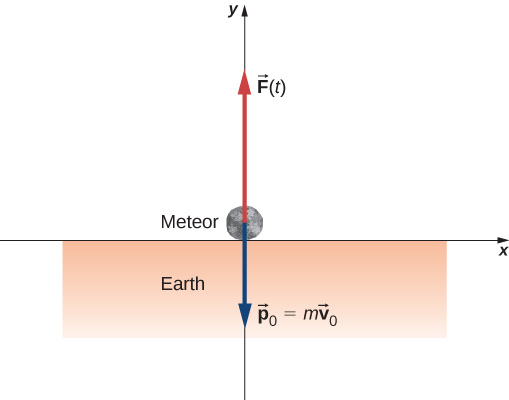The average force during the impact is related to the impulse by

${\mathbf{\overset{\to }{F}}}_{\text{ave}}=\frac{\mathbf{\overset{\to }{J}}}{\Delta t}.$

From Figure, $\mathbf{\overset{\to }{J}}=m\Delta \mathbf{\overset{\to }{v}}$, so we have

${\mathbf{\overset{\to }{F}}}_{\text{ave}}=\frac{m\Delta \mathbf{\overset{\to }{v}}}{\Delta t}.$

The mass is equal to the product of the meteor’s density and its volume:

$m=\rho V.$

If we assume (guess) that the meteor was roughly spherical, we have

$V=\frac{4}{3}\pi {R}^{3}.$

Thus we obtain

${\mathbf{\overset{\to }{F}}}_{\text{ave}}=\frac{\rho V\Delta \mathbf{\overset{\to }{v}}}{\Delta t}=\frac{\rho (\frac{4}{3}\pi {R}^{3})({\mathbf{\overset{\to }{v}}}_{\text{f}}-{\mathbf{\overset{\to }{v}}}_{\text{i}})}{\Delta t}.$

The problem says the velocity at impact was $-1.28\times {10}^{4}\,\text{m/s}\mathbf{\hat{j}}$ (the final velocity is zero); also, we guess that the primary impact lasted about ${t}_{\text{max}}=2\,\text{s}$. Substituting these values gives

$\begin{array}{cc} \hfill {\mathbf{\overset{\to }{F}}}_{\text{ave}}& =\frac{(7970\,\frac{\text{kg}}{{\text{m}}^{3}})[\frac{4}{3}\pi {(25\,\text{m})}^{3}][0\,\frac{\text{m}}{\text{s}}-(-1.28\times {10}^{4}\,\frac{\text{m}}{\text{s}}\mathbf{\hat{j}})]}{2\,\text{s}}\hfill \\ & =+(3.33\times {10}^{12}\,\text{N})\mathbf{\hat{j}}\hfill \end{array}.$

This is the average force applied during the collision. Notice that this force vector points in the same direction as the change of velocity vector $\Delta \mathbf{\overset{\to }{v}}$.

Next, we calculate the maximum force. The impulse is related to the force function by

$\mathbf{\overset{\to }{J}}={\int }_{{t}_{\text{i}}}^{{t}_{\text{max}}}\mathbf{\overset{\to }{F}}(t)dt.$

We need to make a reasonable choice for the force as a function of time. We define $t=0$ to be the moment the meteor first touches the ground. Then we assume the force is a maximum at impact, and rapidly drops to zero. A function that does this is

$F(t)={F}_{\text{max}}{e}^{\text{−}{t}^{2}\text{/}(2{\tau }^{2})}.$

(The parameter $\tau$ represents how rapidly the force decreases to zero.) The average force is

${F}_{\text{ave}}=\frac{1}{\Delta t}{\int }_{0}^{{t}_{\text{max}}}{F}_{\text{max}}{e}^{\text{−}{t}^{2}\text{/}(2{\tau }^{2})}dt$

where $\Delta t={t}_{\text{max}}-0\,\text{s}$. Since we already have a numeric value for ${F}_{\text{ave}}$, we can use the result of the integral to obtain ${F}_{\text{max}}$.

Choosing $\tau =\frac{1}{e}{t}_{\text{max}}$ (this is a common choice, as you will see in later chapters), and guessing that ${t}_{\text{max}}=2\,\text{s}$, this integral evaluates to

${F}_{\text{avg}}=0.458\,{F}_{\text{max}}.$

Thus, the maximum force has a magnitude of

$\begin{array}{ccc}\hfill 0.458{F}_{\text{max}}& =\hfill & 3.33\times {10}^{12}\,\text{N}\hfill \\ \hfill {F}_{\text{max}}& =\hfill & 7.27\times {10}^{12}\,\text{N}\hfill \end{array}.$

The complete force function, including the direction, is

$\mathbf{\overset{\to }{F}}(t)=(7.27\times {10}^{12}\,\text{N}){e}^{\text{−}{t}^{2}\text{/}(8{\text{s}}^{2})}\mathbf{\hat{y}}.$

This is the force Earth applied to the meteor; by Newton’s third law, the force the meteor applied to Earth is

$\mathbf{\overset{\to }{F}}(t)=\text{−}(7.27\times {10}^{12}\,\text{N}){e}^{\text{−}{t}^{2}\text{/}(8{\text{s}}^{2})}\mathbf{\hat{y}}$

which is the answer to the original question.

#### Significance

The graph of this function contains important information. Let’s graph (the magnitude of) both this function and the average force together (Figure).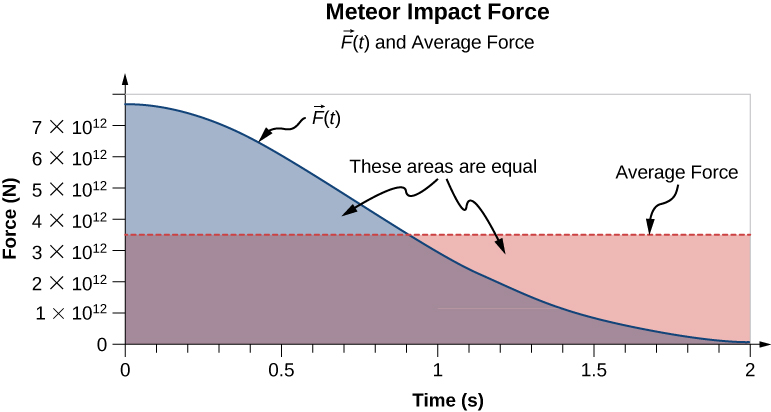Figure 9.8 A graph of the average force (in red) and the force as a function of time (blue) of the meteor impact. The areas under the curves are equal to each other, and are numerically equal to the applied impulse.

Notice that the area under each plot has been filled in. For the plot of the (constant) force ${F}_{\text{ave}}$, the area is a rectangle, corresponding to ${F}_{\text{ave}}\Delta t=J$. As for the plot of F(t), recall from calculus that the area under the plot of a function is numerically equal to the integral of that function, over the specified interval; so here, that is ${\int }_{0}^{{t}_{\text{max}}}F(t)dt=J$. Thus, the areas are equal, and both represent the impulse that the meteor applied to Earth during the two-second impact. The average force on Earth sounds like a huge force, and it is. Nevertheless, Earth barely noticed it. The acceleration Earth obtained was just

$\mathbf{\overset{\to }{a}}=\frac{\text{−}{\mathbf{\overset{\to }{F}}}_{\text{ave}}}{{M}_{\text{Earth}}}=\frac{\text{−}(3.33\times {10}^{12}\,\text{N})\mathbf{\hat{j}}}{5.97\times {10}^{24}\,\text{kg}}=\text{−}(5.6\times {10}^{-13}\,\frac{\text{m}}{{\text{s}}^{2}})\mathbf{\hat{j}}$

which is completely immeasurable. That said, the impact created seismic waves that nowadays could be detected by modern monitoring equipment.

### Example

#### The Benefits of Impulse

A car traveling at 27 m/s collides with a building. The collision with the building causes the car to come to a stop in approximately 1 second. The driver, who weighs 860 N, is protected by a combination of a variable-tension seatbelt and an airbag (Figure). (In effect, the driver collides with the seatbelt and airbag and not with the building.) The airbag and seatbelt slow his velocity, such that he comes to a stop in approximately 2.5 s.

1. What average force does the driver experience during the collision?
2. Without the seatbelt and airbag, his collision time (with the steering wheel) would have been approximately 0.20 s. What force would he experience in this case?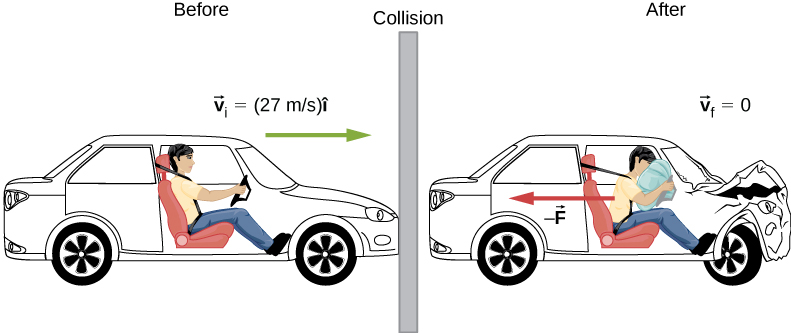Figure 9.9 The motion of a car and its driver at the instant before and the instant after colliding with the wall. The restrained driver experiences a large backward force from the seatbelt and airbag, which causes his velocity to decrease to zero. (The forward force from the seatback is much smaller than the backward force, so we neglect it in the solution.)

#### Strategy

We are given the driver’s weight, his initial and final velocities, and the time of collision; we are asked to calculate a force. Impulse seems the right way to tackle this; we can combine Figure and Figure.

#### Solution

1. Define the +x-direction to be the direction the car is initially moving. We know
$\mathbf{\overset{\to }{J}}=\mathbf{\overset{\to }{F}}\Delta t$

and

$\mathbf{\overset{\to }{J}}=m\Delta \mathbf{\overset{\to }{v}}.$

Since J is equal to both those things, they must be equal to each other:

$\mathbf{\overset{\to }{F}}\Delta t=m\Delta \mathbf{\overset{\to }{v}}.$

We need to convert this weight to the equivalent mass, expressed in SI units:

$\frac{860\,\text{N}}{9.8\,{\text{m/s}}^{2}}=87.8\,\text{kg}.$

Remembering that $\Delta \mathbf{\overset{\to }{v}}={\mathbf{\overset{\to }{v}}}_{\text{f}}-{\mathbf{\overset{\to }{v}}}_{\text{i}}$, and noting that the final velocity is zero, we solve for the force:

$\mathbf{\overset{\to }{F}}=m\frac{0-{v}_{\text{i}}\mathbf{\hat{i}}}{\Delta t}=(87.8\,\text{kg})(\frac{\text{−}(27\,\text{m}\text{/}\text{s})\mathbf{\hat{i}}}{2.5\,\text{s}})=\text{−}(948\,\text{N})\mathbf{\hat{i}}.$

The negative sign implies that the force slows him down. For perspective, this is about 1.1 times his own weight.

2. Same calculation, just the different time interval:
$\mathbf{\overset{\to }{F}}=(87.8\,\text{kg})(\frac{\text{−}(27\,\text{m}\text{/}\text{s})\mathbf{\hat{i}}}{0.20\,\text{s}})=\text{−}(11,853\,\text{N})\mathbf{\hat{i}}$

which is about 14 times his own weight. Big difference!

#### Significance

You see that the value of an airbag is how greatly it reduces the force on the vehicle occupants. For this reason, they have been required on all passenger vehicles in the United States since 1991, and have been commonplace throughout Europe and Asia since the mid-1990s. The change of momentum in a crash is the same, with or without an airbag; the force, however, is vastly different.

### Effect of Impulse

Since an impulse is a force acting for some amount of time, it causes an object’s motion to change. Recall Figure:

$\mathbf{\overset{\to }{J}}=m\Delta \mathbf{\overset{\to }{v}}.$

Because $m\mathbf{\overset{\to }{v}}$ is the momentum of a system, $m\Delta \mathbf{\overset{\to }{v}}$ is the change of momentum $\Delta \mathbf{\overset{\to }{p}}$. This gives us the following relation, called the impulse-momentum theorem (or relation).

#### Impulse-Momentum Theorem

An impulse applied to a system changes the system’s momentum, and that change of momentum is exactly equal to the impulse that was applied:

$\mathbf{\overset{\to }{J}}=\Delta \mathbf{\overset{\to }{p}}.$

The impulse-momentum theorem is depicted graphically in Figure.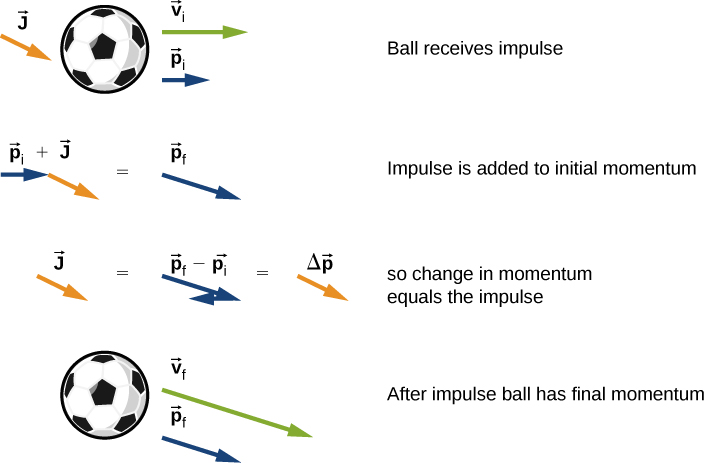Figure 9.10 Illustration of impulse-momentum theorem. (a) A ball with initial velocity ${\mathbf{\overset{\to }{v}}}_{0}$ and momentum ${\mathbf{\overset{\to }{p}}}_{0}$ receives an impulse $\mathbf{\overset{\to }{J}}$. (b) This impulse is added vectorially to the initial momentum. (c) Thus, the impulse equals the change in momentum, $\mathbf{\overset{\to }{J}}=\Delta \mathbf{\overset{\to }{p}}$. (d) After the impulse, the ball moves off with its new momentum ${\mathbf{\overset{\to }{p}}}_{\text{f}}.$

There are two crucial concepts in the impulse-momentum theorem:

1. Impulse is a vector quantity; an impulse of, say, $\text{−}(10\,\text{N}\cdot \text{s})\mathbf{\hat{i}}$ is very different from an impulse of $+(10\,\text{N}\cdot \text{s})\mathbf{\hat{i}}$; they cause completely opposite changes of momentum.
2. An impulse does not cause momentum; rather, it causes a change in the momentum of an object. Thus, you must subtract the final momentum from the initial momentum, and—since momentum is also a vector quantity—you must take careful account of the signs of the momentum vectors.

The most common questions asked in relation to impulse are to calculate the applied force, or the change of velocity that occurs as a result of applying an impulse. The general approach is the same.

#### Problem-Solving Strategy: Impulse-Momentum Theorem

1. Express the impulse as force times the relevant time interval.
2. Express the impulse as the change of momentum, usually $m\Delta v$.
3. Equate these and solve for the desired quantity.

### Example

#### Moving the Enterprise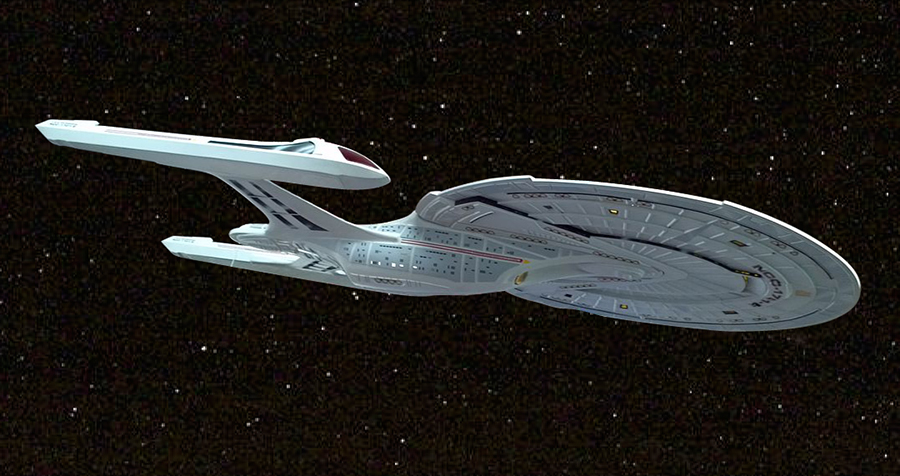Figure 9.11 The fictional starship Enterprise from the Star Trek adventures operated on so-called “impulse engines” that combined matter with antimatter to produce energy.

“Mister Sulu, take us out; ahead one-quarter impulse.” With this command, Captain Kirk of the starship Enterprise (Figure) has his ship start from rest to a final speed of ${v}_{\text{f}}=1\text{/}4(3.0\times {10}^{8}\,\text{m/s})$. Assuming this maneuver is completed in 60 s, what average force did the impulse engines apply to the ship?

#### Strategy

We are asked for a force; we know the initial and final speeds (and hence the change in speed), and we know the time interval over which this all happened. In particular, we know the amount of time that the force acted. This suggests using the impulse-momentum relation. To use that, though, we need the mass of the Enterprise. An internet search gives a best estimate of the mass of the Enterprise (in the 2009 movie) as $2\times {10}^{9}\,\text{kg}$.

#### Solution

Because this problem involves only one direction (i.e., the direction of the force applied by the engines), we only need the scalar form of the impulse-momentum theorem Figure, which is

$\Delta p=J$

with

$\Delta p=m\Delta v$

and

$J=F\Delta t.$

Equating these expressions gives

$F\Delta t=m\Delta v.$

Solving for the magnitude of the force and inserting the given values leads to

$F=\frac{m\Delta v}{\Delta t}=\frac{(2\times {10}^{9}\,\text{kg})(7.5\times {10}^{7}\,\text{m/s})}{60\,\text{s}}=2.5\times {10}^{15}\,\text{N}.$

#### Significance

This is an unimaginably huge force. It goes almost without saying that such a force would kill everyone on board instantly, as well as destroying every piece of equipment. Fortunately, the Enterprise has “inertial dampeners.” It is left as an exercise for the reader’s imagination to determine how these work.

The U.S. Air Force uses “10gs” (an acceleration equal to $10\times 9.8\,{\text{m/s}}^{2}$) as the maximum acceleration a human can withstand (but only for several seconds) and survive. How much time must the Enterprise spend accelerating if the humans on board are to experience an average of at most 10gs of acceleration? (Assume the inertial dampeners are offline.)

Show Solution

To reach a final speed of ${v}_{\text{f}}=\frac{1}{4}(3.0\times {10}^{8}\,\text{m/s})$ at an acceleration of 10g, the time

required is

$\begin{array}{ccc}\hfill 10g& =\hfill & \frac{{v}_{\text{f}}}{\Delta t}\hfill \\ \hfill \Delta t& =\hfill & \frac{{v}_{\text{f}}}{10g}\,\frac{\frac{1}{4}(3.0\times {10}^{8}\,\text{m/s})}{10g}=7.7\times {10}^{5}\,\text{s}=8.9\,\text{d}\hfill \end{array}$

### Example

#### The iPhone Drop

Apple released its iPhone 6 Plus in November 2014. According to many reports, it was originally supposed to have a screen made from sapphire, but that was changed at the last minute for a hardened glass screen. Reportedly, this was because the sapphire screen cracked when the phone was dropped. What force did the iPhone 6 Plus experience as a result of being dropped?

#### Strategy

The force the phone experiences is due to the impulse applied to it by the floor when the phone collides with the floor. Our strategy then is to use the impulse-momentum relationship. We calculate the impulse, estimate the impact time, and use this to calculate the force.

We need to make a couple of reasonable estimates, as well as find technical data on the phone itself. First, let’s suppose that the phone is most often dropped from about chest height on an average-height person. Second, assume that it is dropped from rest, that is, with an initial vertical velocity of zero. Finally, we assume that the phone bounces very little—the height of its bounce is assumed to be negligible.

#### Solution

Define upward to be the +y-direction. A typical height is approximately $h=1.5\,\text{m}$ and, as stated, ${\mathbf{\overset{\to }{v}}}_{\text{i}}=(0\,\text{m/s})\mathbf{\hat{i}}$. The average force on the phone is related to the impulse the floor applies on it during the collision:

${\mathbf{\overset{\to }{F}}}_{\text{ave}}=\frac{\mathbf{\overset{\to }{J}}}{\Delta t}.$

The impulse $\mathbf{\overset{\to }{J}}$ equals the change in momentum,

$\mathbf{\overset{\to }{J}}=\Delta \mathbf{\overset{\to }{p}}$

so

${\mathbf{\overset{\to }{F}}}_{\text{ave}}=\frac{\Delta \mathbf{\overset{\to }{p}}}{\Delta t}.$

Next, the change of momentum is

$\Delta \mathbf{\overset{\to }{p}}=m\Delta \mathbf{\overset{\to }{v}}.$

We need to be careful with the velocities here; this is the change of velocity due to the collision with the floor. But the phone also has an initial drop velocity ${\mathbf{\overset{\to }{v}}}_{\text{i}}=(0\,\text{m/s})\mathbf{\hat{j}}$, so we label our velocities. Let:

• ${\mathbf{\overset{\to }{v}}}_{\text{i}}=$ the initial velocity with which the phone was dropped (zero, in this example)
• ${\mathbf{\overset{\to }{v}}}_{1}=$ the velocity the phone had the instant just before it hit the floor
• ${\mathbf{\overset{\to }{v}}}_{2}=$ the final velocity of the phone as a result of hitting the floor

Figure shows the velocities at each of these points in the phone’s trajectory.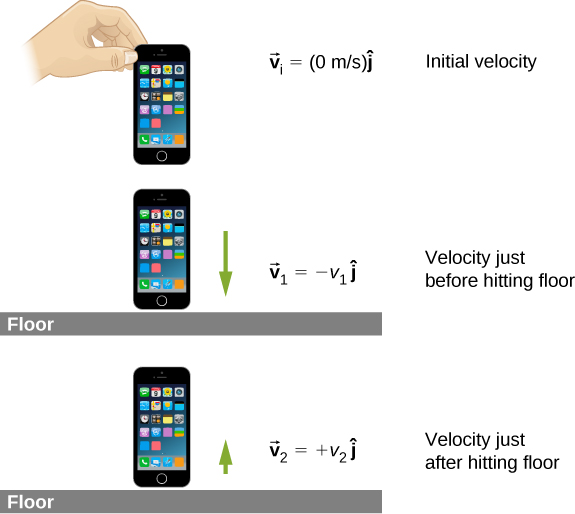Figure 9.12 (a) The initial velocity of the phone is zero, just after the person drops it. (b) Just before the phone hits the floor, its velocity is ${\mathbf{\overset{\to }{v}}}_{1},$ which is unknown at the moment, except for its direction, which is downward $(\text{−}\mathbf{\hat{j}}).$ (c) After bouncing off the floor, the phone has a velocity ${\mathbf{\overset{\to }{v}}}_{2}$, which is also unknown, except for its direction, which is upward $(+\mathbf{\hat{j}}).$

With these definitions, the change of momentum of the phone during the collision with the floor is

$m\Delta \mathbf{\overset{\to }{v}}=m({\mathbf{\overset{\to }{v}}}_{2}-{\mathbf{\overset{\to }{v}}}_{1}).$

Since we assume the phone doesn’t bounce at all when it hits the floor (or at least, the bounce height is negligible), then ${\mathbf{\overset{\to }{v}}}_{2}$ is zero, so

$\begin{array}{ccc}\hfill m\Delta \mathbf{\overset{\to }{v}}& =\hfill & m[0-(\text{−}{v}_{1}\mathbf{\hat{j}})]\hfill \\ \hfill m\Delta \mathbf{\overset{\to }{v}}& =\hfill & +m{v}_{1}\mathbf{\hat{j}}.\hfill \end{array}$

We can get the speed of the phone just before it hits the floor using either kinematics or conservation of energy. We’ll use conservation of energy here; you should re-do this part of the problem using kinematics and prove that you get the same answer.

First, define the zero of potential energy to be located at the floor. Conservation of energy then gives us:

$\begin{array}{ccc}\hfill {E}_{\text{i}}& =\hfill & {E}_{1}\hfill \\ \hfill {K}_{\text{i}}+{U}_{\text{i}}& =\hfill & {K}_{1}+{U}_{1}\hfill \\ \hfill \frac{1}{2}m{v}_{\text{i}}^{2}+mg{h}_{\text{drop}}& =\hfill & \frac{1}{2}m{v}_{1}^{2}+mg{h}_{\text{floor}}.\hfill \end{array}$

Defining ${h}_{\text{floor}}=0$ and using ${\mathbf{\overset{\to }{v}}}_{\text{i}}=(0\,\text{m/s})\mathbf{\hat{j}}$ gives

$\begin{array}{ccc}\hfill \frac{1}{2}m{v}_{1}^{2}& =\hfill & mg{h}_{\text{drop}}\hfill \\ \hfill {v}_{1}& =\hfill & \pm\sqrt{2g{h}_{\text{drop}}}.\hfill \end{array}$

Because ${v}_{1}$ is a vector magnitude, it must be positive. Thus, $m\Delta v=m{v}_{1}=m\sqrt{2g{h}_{\text{drop}}}$. Inserting this result into the expression for force gives

$\begin{array}{cc}\hfill \mathbf{\overset{\to }{F}}& =\frac{\Delta \mathbf{\overset{\to }{p}}}{\Delta t}\hfill \\ & =\frac{m\Delta \mathbf{\overset{\to }{v}}}{\Delta t}\hfill \\ & =\frac{+m{v}_{1}\mathbf{\hat{j}}}{\Delta t}\hfill \\ & =\frac{m\sqrt{2gh}}{\Delta t}\mathbf{\hat{j}}.\hfill \end{array}$

Finally, we need to estimate the collision time. One common way to estimate a collision time is to calculate how long the object would take to travel its own length. The phone is moving at 5.4 m/s just before it hits the floor, and it is 0.14 m long, giving an estimated collision time of 0.026 s. Inserting the given numbers, we obtain

$\mathbf{\overset{\to }{F}}=\frac{(0.172\,\text{kg})\sqrt{2(9.8\,{\text{m/s}}^{2})(1.5\,\text{m})}}{0.026\,\text{s}}\mathbf{\hat{j}}=(36\,\text{N})\mathbf{\hat{j}}.$

#### Significance

The iPhone itself weighs just $(0.172\,\text{kg})(9.81\,{\text{m/s}}^{2})=1.68\,\text{N}$; the force the floor applies to it is therefore over 20 times its weight.

What if we had assumed the phone did bounce on impact? Would this have increased the force on the iPhone, decreased it, or made no difference?

Show Solution

If the phone bounces up with approximately the same initial speed as its impact speed, the change in momentum of the phone will be $\Delta \mathbf{\overset{\to }{p}}=m\Delta \mathbf{\overset{\to }{v}}-(\text{−}m\Delta \mathbf{\overset{\to }{v}})=2m\Delta \mathbf{\overset{\to }{v}}$. This is twice the momentum change than when the phone does not bounce, so the impulse-momentum theorem tells us that more force must be applied to the phone.

### Momentum and Force

In Figure, we obtained an important relationship:

${\mathbf{\overset{\to }{F}}}_{\text{ave}}=\frac{\Delta \mathbf{\overset{\to }{p}}}{\Delta t}.$

In words, the average force applied to an object is equal to the change of the momentum that the force causes, divided by the time interval over which this change of momentum occurs. This relationship is very useful in situations where the collision time $\Delta t$ is small, but measureable; typical values would be 1/10th of a second, or even one thousandth of a second. Car crashes, punting a football, or collisions of subatomic particles would meet this criterion.

For a continuously changing momentum—due to a continuously changing force—this becomes a powerful conceptual tool. In the limit $\Delta t\to dt$, Figure becomes

$\mathbf{\overset{\to }{F}}=\frac{d\mathbf{\overset{\to }{p}}}{dt}.$

This says that the rate of change of the system’s momentum (implying that momentum is a function of time) is exactly equal to the net applied force (also, in general, a function of time). This is, in fact, Newton’s second law, written in terms of momentum rather than acceleration. This is the relationship Newton himself presented in his Principia Mathematica (although he called it “quantity of motion” rather than “momentum”).

If the mass of the system remains constant, Figure reduces to the more familiar form of Newton’s second law. We can see this by substituting the definition of momentum:

$\mathbf{\overset{\to }{F}}=\frac{d(m\mathbf{\overset{\to }{v}})}{dt}=m\frac{d\mathbf{\overset{\to }{v}}}{dt}=m\mathbf{\overset{\to }{a}}.$

The assumption of constant mass allowed us to pull m out of the derivative. If the mass is not constant, we cannot use this form of the second law, but instead must start from Figure. Thus, one advantage to expressing force in terms of changing momentum is that it allows for the mass of the system to change, as well as the velocity; this is a concept we’ll explore when we study the motion of rockets.

#### Newton’s Second Law of Motion in Terms of Momentum

The net external force on a system is equal to the rate of change of the momentum of that system caused by the force:

$\mathbf{\overset{\to }{F}}=\frac{d\mathbf{\overset{\to }{p}}}{dt}.$

Although Figure allows for changing mass, as we will see in Rocket Propulsion, the relationship between momentum and force remains useful when the mass of the system is constant, as in the following example.

### Example

#### Calculating Force: Venus Williams’ Tennis Serve

During the 2007 French Open, Venus Williams hit the fastest recorded serve in a premier women’s match, reaching a speed of 58 m/s (209 km/h). What is the average force exerted on the 0.057-kg tennis ball by Venus Williams’ racquet? Assume that the ball’s speed just after impact is 58 m/s, as shown in Figure, that the initial horizontal component of the velocity before impact is negligible, and that the ball remained in contact with the racquet for 5.0 ms.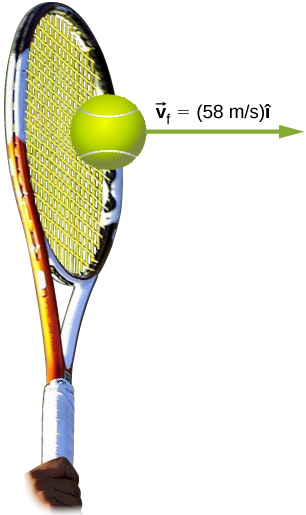Figure 9.13 The final velocity of the tennis ball is ${\mathbf{\overset{\to }{v}}}_{\text{f}}=(58\,\text{m/s})\mathbf{\hat{i}}$.

#### Strategy

This problem involves only one dimension because the ball starts from having no horizontal velocity component before impact. Newton’s second law stated in terms of momentum is then written as

$\mathbf{\overset{\to }{F}}=\frac{d\mathbf{\overset{\to }{p}}}{dt}.$

As noted above, when mass is constant, the change in momentum is given by

$\Delta p=m\Delta v=m({v}_{\text{f}}-{v}_{\text{i}})$

where we have used scalars because this problem involves only one dimension. In this example, the velocity just after impact and the time interval are given; thus, once $\Delta p$ is calculated, we can use$F=\frac{\Delta p}{\Delta t}$ to find the force.

#### Solution

To determine the change in momentum, insert the values for the initial and final velocities into the equation above:

$\begin{array}{cc}\hfill \Delta p& =m({v}_{\text{f}}-{v}_{\text{i}})\hfill \\ & =(0.057\,\text{kg})(58\,\text{m/s}-\,0\,\text{m/s})\hfill \\ & =3.3\,\frac{\text{kg}\cdot \text{m}}{\text{s}}.\hfill \end{array}$

Now the magnitude of the net external force can be determined by using

$F=\frac{\Delta p}{\Delta t}=\frac{3.3\,\frac{\text{kg}\cdot \text{m}}{\text{s}}}{5.0\times {10}^{-3}\,\text{s}}=6.6\times {10}^{2}\,\text{N.}$

where we have retained only two significant figures in the final step.

#### Significance

This quantity was the average force exerted by Venus Williams’ racquet on the tennis ball during its brief impact (note that the ball also experienced the 0.57-N force of gravity, but that force was not due to the racquet). This problem could also be solved by first finding the acceleration and then using $F=ma$, but one additional step would be required compared with the strategy used in this example.

### Summary

• When a force is applied on an object for some amount of time, the object experiences an impulse.
• This impulse is equal to the object’s change of momentum.
• Newton’s second law in terms of momentum states that the net force applied to a system equals the rate of change of the momentum that the force causes.

### Conceptual Questions

Is it possible for a small force to produce a larger impulse on a given object than a large force? Explain.

Show Solution

Yes; impulse is the force applied multiplied by the time during which it is applied ($J=F\Delta t$), so if a small force acts for a long time, it may result in a larger impulse than a large force acting for a small time.

Why is a 10-m fall onto concrete far more dangerous than a 10-m fall onto water?

What external force is responsible for changing the momentum of a car moving along a horizontal road?

Show Solution

By friction, the road exerts a horizontal force on the tires of the car, which changes the momentum of the car.

A piece of putty and a tennis ball with the same mass are thrown against a wall with the same velocity. Which object experience a greater impulse from the wall or are the impulses equal? Explain.

### Problems

A 75.0-kg person is riding in a car moving at 20.0 m/s when the car runs into a bridge abutment (see the following figure).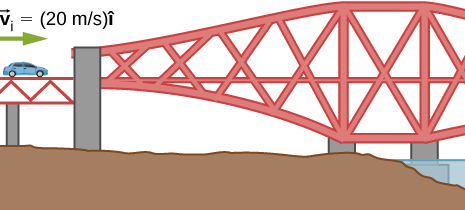1. Calculate the average force on the person if he is stopped by a padded dashboard that compresses an average of 1.00 cm.
2. Calculate the average force on the person if he is stopped by an air bag that compresses an average of 15.0 cm.
Show Solution

a. $1.50\times {10}^{6}\,\text{N}$; b. $1.00\times {10}^{5}\,\text{N}$

One hazard of space travel is debris left by previous missions. There are several thousand objects orbiting Earth that are large enough to be detected by radar, but there are far greater numbers of very small objects, such as flakes of paint. Calculate the force exerted by a 0.100-mg chip of paint that strikes a spacecraft window at a relative speed of $4.00\times {10}^{3}\,\text{m/s}$, given the collision lasts $6.00\times {10}^{-8}\,\text{s}$.

A cruise ship with a mass of $1.00\times {10}^{7}\,\text{kg}$ strikes a pier at a speed of 0.750 m/s. It comes to rest after traveling 6.00 m, damaging the ship, the pier, and the tugboat captain’s finances. Calculate the average force exerted on the pier using the concept of impulse. (Hint: First calculate the time it took to bring the ship to rest, assuming a constant force.)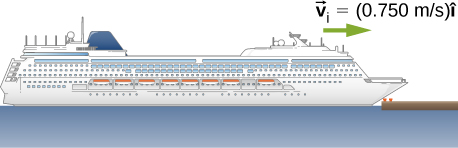Show Solution

$4.69\times {10}^{5}\,\text{N}$

Calculate the final speed of a 110-kg rugby player who is initially running at 8.00 m/s but collides head-on with a padded goalpost and experiences a backward force of $1.76\times {10}^{4}\,\text{N}$ for $5.50\times {10}^{-2}\,\text{s}$.

Water from a fire hose is directed horizontally against a wall at a rate of 50.0 kg/s and a speed of 42.0 m/s. Calculate the force exerted on the wall, assuming the water’s horizontal momentum is reduced to zero.

Show Solution

$2.10\times {10}^{3}\,\text{N}$

A 0.450-kg hammer is moving horizontally at 7.00 m/s when it strikes a nail and comes to rest after driving the nail 1.00 cm into a board. Assume constant acceleration of the hammer-nail pair.

1. Calculate the duration of the impact.
2. What was the average force exerted on the nail?

What is the momentum (as a function of time) of a 5.0-kg particle moving with a velocity $\mathbf{\overset{\to }{v}}(t)=(2.0\mathbf{\hat{i}}+4.0t\mathbf{\hat{j}})\,\text{m/s}$? What is the net force acting on this particle?

Show Solution

$\mathbf{\overset{\to }{p}}(t)=(10\mathbf{\hat{i}}+20t\mathbf{\hat{j}})\text{kg}\cdot \text{m}\text{/}\text{s}$;$\mathbf{\overset{\to }{F}}=(20\,\text{N})\mathbf{\hat{j}}$

The x-component of a force on a 46-g golf ball by a 7-iron versus time is plotted in the following figure: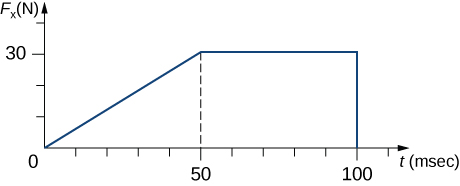1. Find the x-component of the impulse during the intervals
1. [0, 50 ms], and
2. [50 ms, 100 ms]
2. Find the change in the x-component of the momentum during the intervals
1. [0, 50 ms], and
2. [50 ms, 100 ms]

A hockey puck of mass 150 g is sliding due east on a frictionless table with a speed of 10 m/s. Suddenly, a constant force of magnitude 5 N and direction due north is applied to the puck for 1.5 s. Find the north and east components of the momentum at the end of the 1.5-s interval.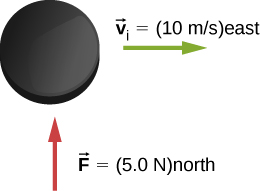Show Solution

Let the positive x-axis be in the direction of the original momentum. Then ${p}_{x}=1.5\,\text{kg}\cdot \text{m/s}$ and ${p}_{y}=7.5\,\text{kg}\cdot \text{m/s}$

A ball of mass 250 g is thrown with an initial velocity of 25 m/s at an angle of $30^\circ$ with the horizontal direction. Ignore air resistance. What is the momentum of the ball after 0.2 s? (Do this problem by finding the components of the momentum first, and then constructing the magnitude and direction of the momentum vector from the components.)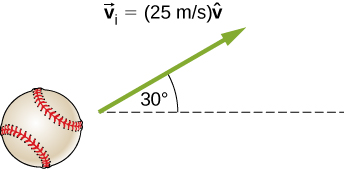### Glossary

impulse
effect of applying a force on a system for a time interval; this time interval is usually small, but does not have to be
impulse-momentum theorem
change of momentum of a system is equal to the impulse applied to the system